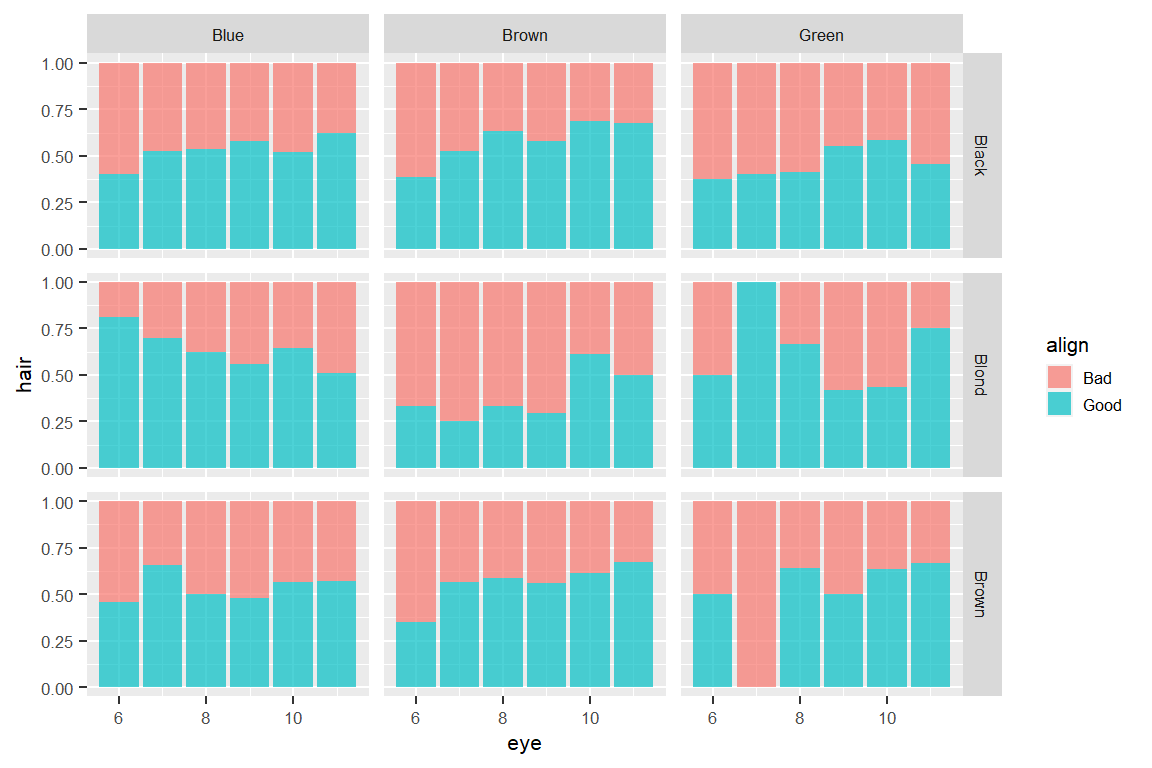🏠 Key Points :
■ 3 different types of bar plots in ggplot
■ Bar plots for group comparison
■ Choose the basis of comparison by setting position
■ Ill-designed bar plots could be misleading in an intuitive way! ⏰

### 1. The three kinds of bars

Load libraries and set global options

pacman::p_load(dplyr,ggplot2,plotly,gridExtra)
theme_set(theme_get() + theme(
text=element_text(size=8), legend.key.size=unit(10,"points")
))

D = read.csv("data/comics1.csv") %>% as_tibble
glimpse(D)
Rows: 7,250
Columns: 9
$publisher <chr> "dc", "dc", "dc", "dc", "dc", "dc", "dc", "dc", "dc", "dc"~$ name        <chr> "Batman (Bruce Wayne)", "Superman (Clark Kent)", "Green La~
align <chr> "Good", "Good", "Good", "Good", "Good", "Good", "Good", "G~ eye         <chr> "Blue", "Blue", "Brown", "Brown", "Blue", "Blue", "Blue", ~
$hair <chr> "Black", "Black", "Brown", "White", "Black", "Black", "Blo~$ sex         <chr> "Male", "Male", "Male", "Male", "Male", "Female", "Male", ~
$alive <chr> "Living", "Living", "Living", "Living", "Living", "Living"~$ appearances <int> 3093, 2496, 1565, 1316, 1237, 1231, 1121, 1095, 1075, 1028~
year <int> 1939, 1986, 1959, 1987, 1940, 1941, 1941, 1989, 1969, 1956~ tibble is an enhanced data frame defined in dplyr. We convert D into tibble for better display. 🌻 There 3 types of bar plots in ggplot • geom_histogram shows the distribution of continuous variables. • geom_bar shows the counts (distribution) of discrete variables. • geom_col shows/compares group statistics. g1 = ggplot(D, aes(x=year)) + geom_histogram(binwidth=10) g2 = ggplot(D, aes(x=align)) + geom_bar() g3 = group_by(D, sex) %>% summarise( casualty.ratio = mean(alive=="Deceased") ) %>% ggplot(aes(x=sex, y=casualty.ratio)) + geom_col() grid.arrange(g1,g2,g3,nrow=1) # align three plots in a row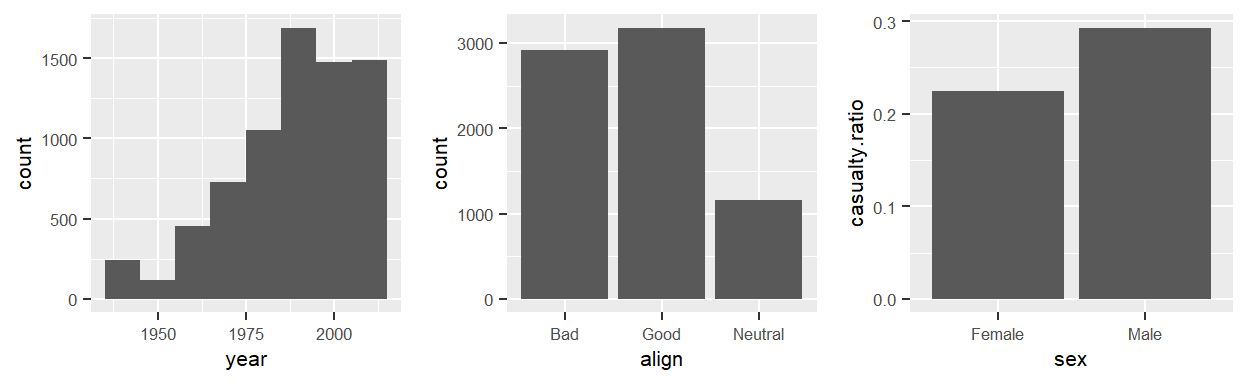🌻 These bar plot help us compare • the numbers of new characters by decades • the numbers of Good, Bad and Neutral characters • Male and Female characters’ average casualty rates ### 2. Sub-Groups in Different Colors The complexity of bar plots kicks in when the comparisons involve sub-groups. To distinguish sub-groups within a bars, we map the fill attribute to the sub-grouping variable. g1 = ggplot(D, aes(x=year, fill=publisher)) + geom_histogram(binwidth=10) g2 = ggplot(D, aes(x=align, fill=sex)) + geom_bar() grid.arrange(g1,g2,nrow=1) # align three plots in a row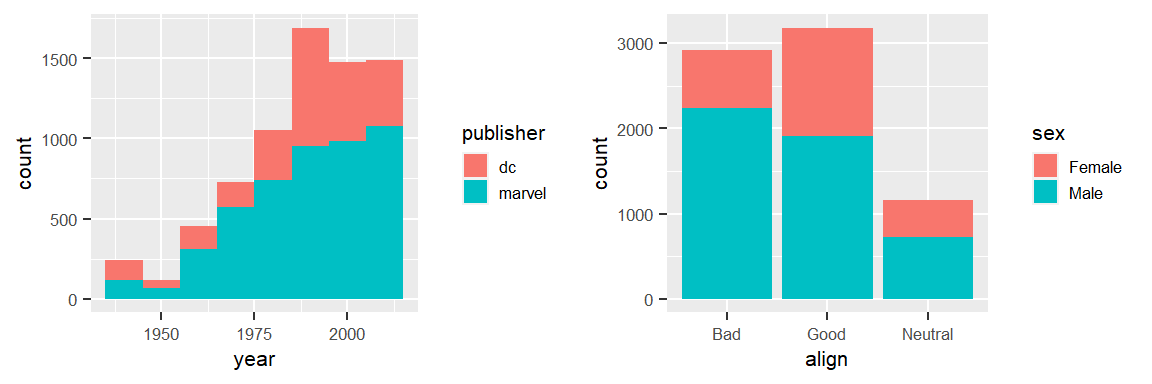🚴 EXERCISE : Try to make the following chart by modifying the code snippet below. # group_by(D, sex) %>% # summarise( casualty.ratio = mean(alive=="Deceased") ) %>% # ggplot(aes(x=sex, y=casualty.ratio)) + geom_col()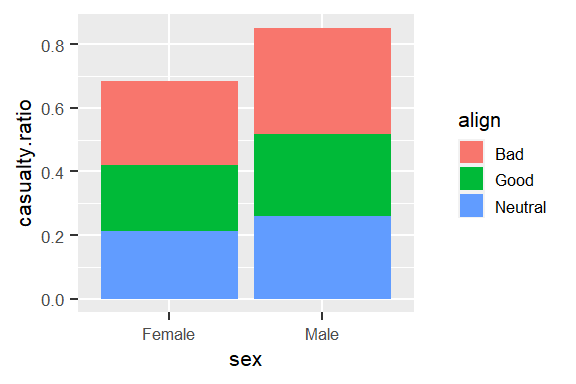DISCUSSION: Based on the chart, above … • What are we comparing among the subgroups? • Is the causality ratio of the bad-males higher than that of the bad-females? • There are a few problems in this chart • It make no sense to add up the casualty.ratios • Stacking makes it difficult to compare among the subgroups We can cope with these problem by using the position argument within geom_col()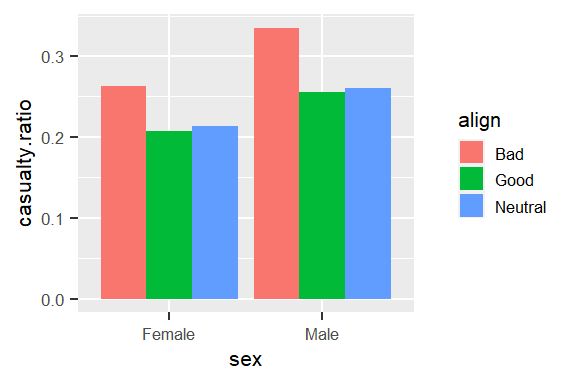See? Now we can compare the casualty ratio of all of the subgroups easily. ### 3. The Foundation of Comparison ##### 3.1 Contingency Table Sub-Group comparison is based on a data structure called Contingency Table. table(Dalign, Dsex)  Female Male Bad 676 2239 Good 1264 1914 Neutral 432 725 ##### 3.2 The Long Format of Contingency Table However, ggplot cannot take the table format. To be compatible with the aes() mapping mechanism, we need to prepare the data in the Long Format. count() is a handy way to make long table, when comparing to group_by() %>% summarise(). count(D, align, sex) # A tibble: 6 x 3 align sex n <chr> <chr> <int> 1 Bad Female 676 2 Bad Male 2239 3 Good Female 1264 4 Good Male 1914 5 Neutral Female 432 6 Neutral Male 725 ##### 3.3 Change the Basis of Comparison by setting position By setting the position argument in geom_col(), we can align and compare the numbers in different ways. dx = count(D, align, sex) gg = lapply(c("stack","dodge","fill"), function(pos) { ggplot(dx, aes(sex,n,fill=align)) + geom_col(position=pos, alpha=0.6) + labs(title=pos,y="") }) grid.arrange(grobs=gg, nrow=1)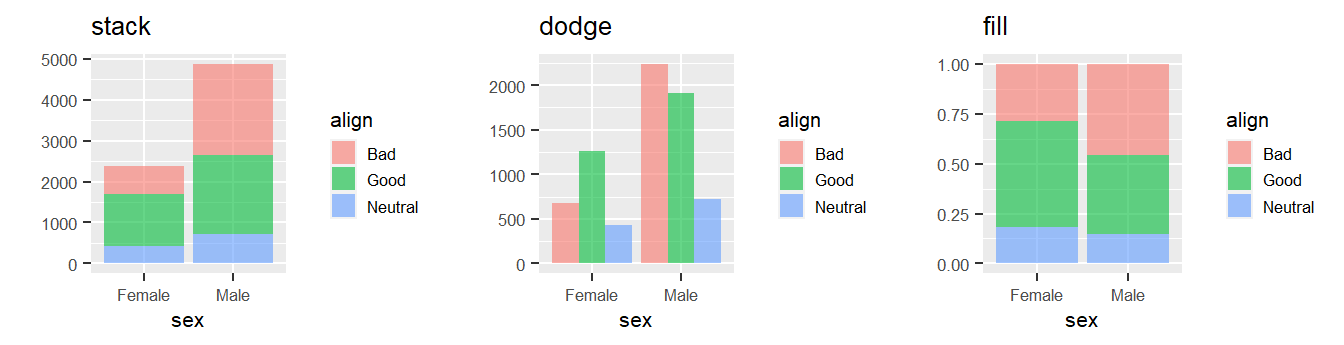🌻 Different plot serves different purpose … • stack the numbers emphasizes the sums by sex • dodge to compare all of the numbers in the table • fill convert numbers into factions for relative comparison QUIZ : Which of the 3 above charts is easier to … • compare the numbers of Neutral by sex • compare the ratio of Bad characters by sex • compare the numbers characters in each sex 🚴 EXERCISE : Actually we can make three more plots out of exactly the same data. Try to make the following chart by modifying the code snippet below. # gg = lapply(c("stack","dodge","fill"), function(pos) { # ggplot(dx, aes(sex,n,fill=align)) + # geom_col(position=pos, alpha=0.6) + labs(title=pos,y="") # }) # grid.arrange(grobs=gg, nrow=1)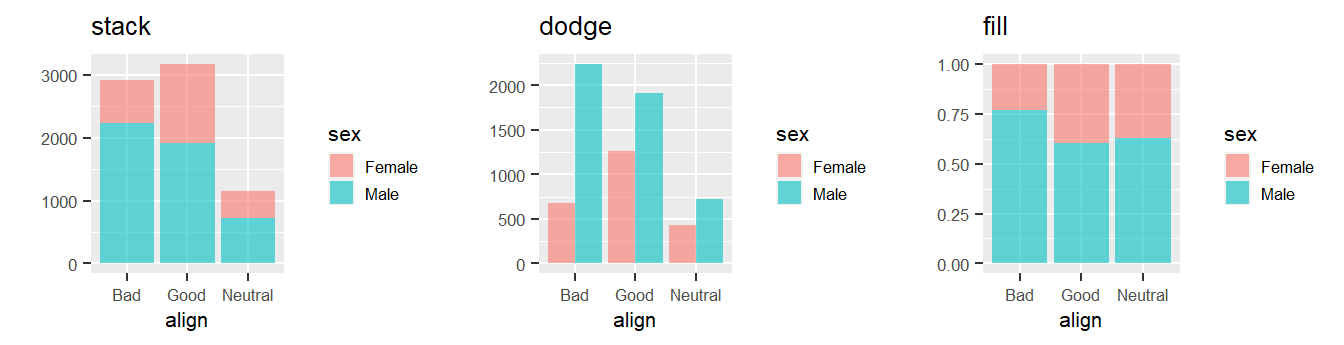### 4. Seeing Trend by Bar Plots Let’s mutate a decade column in D. D = mutate(D, decade = (Dyear -1900) %/% 10)
table(Ddecade)  3 4 5 6 7 8 9 10 11 28 271 106 678 823 1304 1581 1803 656  ❓ How is the number of each align varies in time by sex, by publisher? ggplot(D, aes(x=decade, fill=align)) + geom_bar() + facet_grid(sex~publisher)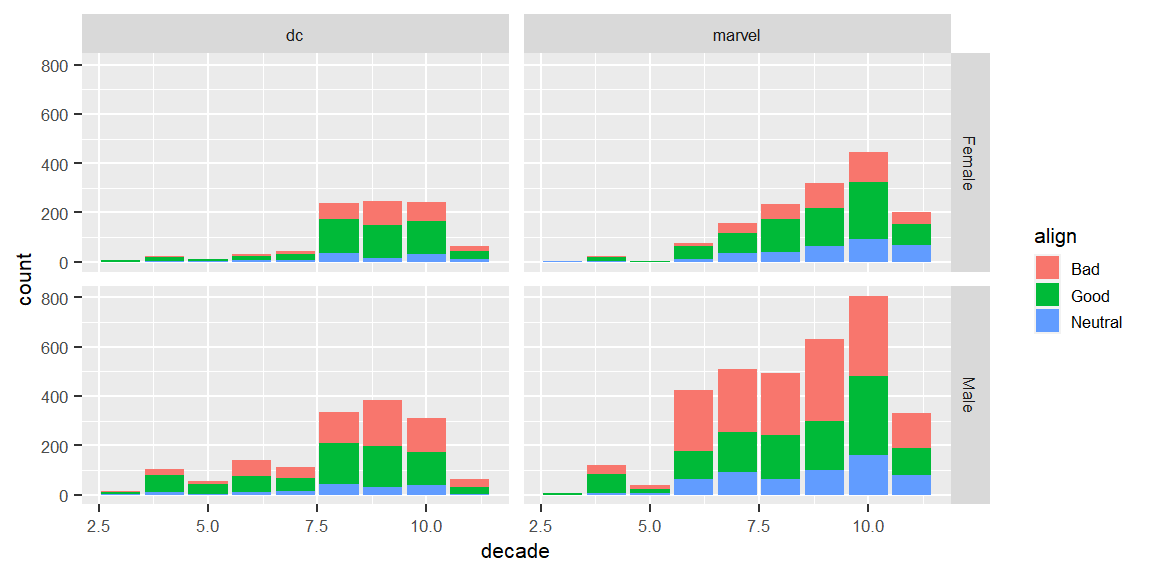🌷 See how easy we can answer this seemingly complicate query in two lines of simple code. If I’d answered this query with a table full of numbers, would it be helpful at all. Comparing to the numbers, the variations in ratios might better reflect the trend. To convert numbers into ratios, we simply put position='fill' in geom_bar(). # here we convert align into a factor so we can # re-order the align levels in a desirable way D2 = D %>% mutate( align=factor(align,levels=c("Bad","Neutral","Good")) ) %>% filter(decade >= 6) ggplot(D2, aes(x=decade, fill=align)) + geom_bar(position="fill") + facet_grid(sex~publisher)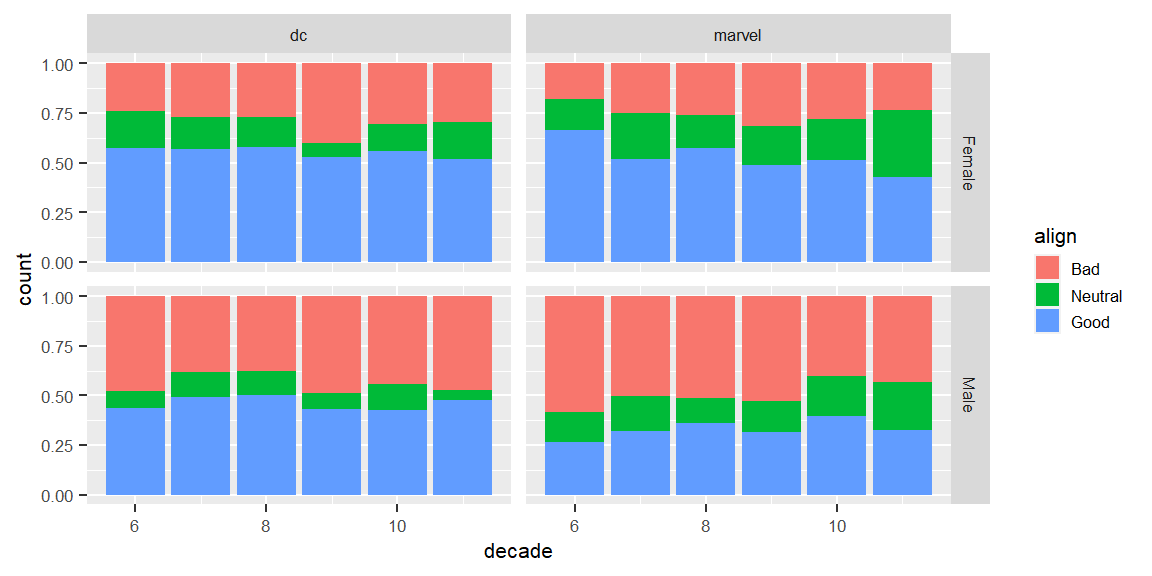Below is a bar plot that show ratios of good and bad aligns in different hair and eye colors. hx = count(D2, hair, sort=T) ex = count(D2, eye, sort=T) D2 %>% filter( hair%in%hxhair[1:3], eye%in%ex\$eye[1:3],
align!="Neutral") %>%
facet_grid(hair~eye)